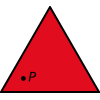#### You may also like### Fitting In

The largest square which fits into a circle is ABCD and EFGH is a square with G and H on the line CD and E and F on the circumference of the circle. Show that AB = 5EF. Similarly the largest equilateral triangle which fits into a circle is LMN and PQR is an equilateral triangle with P and Q on the line LM and R on the circumference of the circle. Show that LM = 3PQ### Triangle Midpoints

You are only given the three midpoints of the sides of a triangle. How can you construct the original triangle?### Three Way Split

Take any point P inside an equilateral triangle. Draw PA, PB and PC from P perpendicular to the sides of the triangle where A, B and C are points on the sides. Prove that PA + PB + PC is a constant.

# Tetrahedra Tester

##### Age 14 to 16Challenge Level
We received the following solution from Ben:

Using the combination nCr,
6C3 = all the possible triangles = 20.
If you take each possible triangle as a "base", then use nPr to find the number of tetrahedra that can be constructed from one base.
3P3 = 6
We must realize that using these figures, one tetrahedron will be listed four times, each time with a different triangle on the base, but still the same tetrahedron. Therefore, we must divide the figure by 4.
The lengths 4, 5 and 9 cannot form a triangle, so tetrahedra with this value must be removed. There are 6 tetrahedra with base 4, 5 and 9, so there 4 times (24) this value listed. Therefore, we must subtract this value from the figure.
6 x 20 = 120 = all hypothetical tetrahedra
120 - 24 = 96 = all possible tetrahedra (inc. tetrahedra counted more than once)
96 / 4 = 24 = total number of tetrahedra that can be made
ans = 24